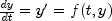JMSLTM Numerical Library 7.2.0
com.imsl.math

## Class OdeRungeKutta

• All Implemented Interfaces:
Serializable, Cloneable

```public class OdeRungeKutta
extends ODE```
Solves an initial-value problem for ordinary differential equations using the Runge-Kutta-Verner fifth-order and sixth-order method.

Class `OdeRungeKutta` finds an approximation to the solution of a system of first-order differential equations of the formwith given initial data. The class attempts to keep the global error proportional to a user-specified tolerance. This class is efficient for nonstiff systems where the derivative evaluations are not expensive.

`OdeRungeKutta` is based on a code designed by Hull, Enright and Jackson (1976, 1977). It uses Runge-Kutta formulas of order five and six developed by J. H. Verner.

Example, Serialized Form
• ### Nested Class Summary

Nested Classes
Modifier and Type Class and Description
`static class ` `OdeRungeKutta.DidNotConvergeException`
The iteration did not converge within the maximum number of steps allowed (default 500).
`static interface ` `OdeRungeKutta.Function`
Public interface for user supplied function to `OdeRungeKutta` object.
`static class ` `OdeRungeKutta.ToleranceTooSmallException`
Tolerance is too small or the problem is stiff.

• ### Fields inherited from class com.imsl.math.ODE

`AFTER_SUCCESSFUL_STEP, AFTER_UNSUCCESSFUL_STEP, BEFORE_STEP, ERROR_NORM_ABS, ERROR_NORM_EUCLIDEAN, ERROR_NORM_MAX, ERROR_NORM_MINABSREL`
• ### Constructor Summary

Constructors
Constructor and Description
`OdeRungeKutta(OdeRungeKutta.Function function)`
Constructs an ODE solver to solve the initial value problem dy/dt = f(t,y)
• ### Method Summary

Methods
Modifier and Type Method and Description
`void` `setMaximumStepsize(double stepsize)`
Sets the maximum internal step size.
`void` ```solve(double t, double tEnd, double[] y)```
Integrates the ODE system from `t` to `tEnd`.
• ### Methods inherited from class com.imsl.math.ODE

`examineStep, getFloor, getInitialStepsize, getMaximumStepsize, getMaxSteps, getMinimumStepsize, getNorm, getScale, getTolerance, setFloor, setInitialStepsize, setMaxSteps, setMinimumStepsize, setNorm, setScale, setTolerance, vnorm`
• ### Methods inherited from class java.lang.Object

`clone, equals, finalize, getClass, hashCode, notify, notifyAll, toString, wait, wait, wait`
• ### Constructor Detail

• #### OdeRungeKutta

`public OdeRungeKutta(OdeRungeKutta.Function function)`
Constructs an ODE solver to solve the initial value problem dy/dt = f(t,y)
Parameters:
`function` - implementation of interface Function that defines the right-hand side function f(t,y)
• ### Method Detail

• #### setMaximumStepsize

`public void setMaximumStepsize(double stepsize)`
Sets the maximum internal step size.
Overrides:
`setMaximumStepsize` in class `ODE`
Parameters:
`stepsize` - a `double` specifying the maximum internal step size. `stepsize` must be greater than zero. Default: `stepsize` = 2
• #### solve

```public void solve(double t,
double tEnd,
double[] y)
throws OdeRungeKutta.ToleranceTooSmallException,
OdeRungeKutta.DidNotConvergeException```
Integrates the ODE system from `t` to `tEnd`. On all but the first call to solve, the value of `t` must equal the value of `tEnd` from the previous call.
Parameters:
`t` - `double` specifying the independent variable
`tEnd` - `double` specifying the value of `t` at which the solution is desired
`y` - on input, `double` array containing the initial values. On output, `double` array containing the approximate solution.
Throws:
`OdeRungeKutta.DidNotConvergeException` - is thrown if the number of internal steps exceeds maxSteps (default 500). This can be an indication that the ODE system is stiff. This exception can also be thrown if the error tolerance condition could not be met.
`OdeRungeKutta.ToleranceTooSmallException` - is thrown if the computation does not converge on some step.
JMSLTM Numerical Library 7.2.0